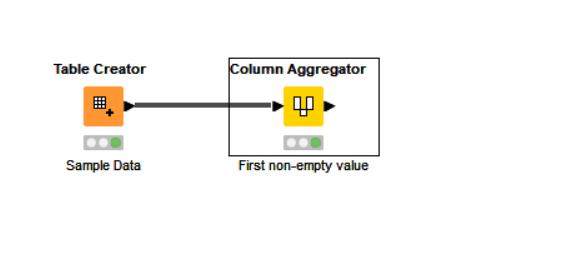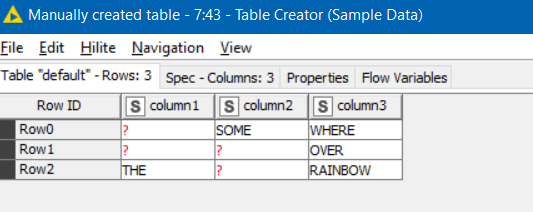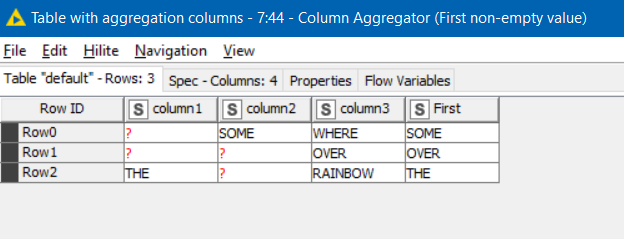# KNIME IF column expression with multiple columns

Hi KNIME Community!

I am somewhat new to knime and I would like to ask for your kind help in the below:

I need basically an equivalent for an iferror function in excel, or an if inside an if but more times, for columns. I made the empty cells to put “x” so it was easier for me to put a function on the cells.

I have put my syntax below as per Javascript in the Column expression node:

What I want KNIME to do is, if the first column has x value then take the value from the next column, but if that has x too than take the next etc. That is what you can see with 3 columns, but it only works for the first column, then everything else remains x…

(In the future I need this with 14 columns in order, I am testing it with tree but doesn’t seem to work.

This is the result, it goes from right to left:

It works for the first time, it takes the vale of the 3rd column from the left i the second is x, but if that is x too it just leaves it as x, eventhough the end “else” has values. (“Taxonomy Id - just FDL”)

Hi @olahkrisz ,

Looking at your column expression, the logic is slightly off.
(I’ll simply refer to the columns as col1, col2, col3, col4 and I’m purposely not using the column expression syntax here)
What you currently have is this:

``````if col1=="x" then use value of col2
else
if col2=="x", then use value of col3
else
if col3=="x", then use value of col4
``````

and so on…

Read that through carefully… you only ever use the value of col2 if col1 is “x” (irrespective of the value of col2)
and you only ever check if col2==“x” if col1 is not “x”

What you should be saying is:

``````if col1=="x" then
if col2=="x" then
if col3=="x" then
col4 (etc)
else
use value of col3
else
use value of col2
else
use value of col1
``````

In terms of if there is a better way to do this, especially when scaling to 14 columns, I would say there probably is, assuming my understanding is correct.

If we can turn your “x” values back into missing values, and the aim is to find the first non-missing value on a particular row, then this can be achieved like this:result…6 Likes

This topic was automatically closed 90 days after the last reply. New replies are no longer allowed.